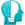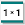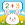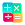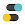# Cool math games: arithmetic & multiplication table

by Standy Software
1,000,000+ installs

Android application Cool math games: arithmetic & multiplication table developed by is listed under category Game Educational. According to Google Play Cool math games: arithmetic & multiplication table achieved more than 1,000,000 installs. Cool math games: arithmetic & multiplication table currently has 5,846 ratings with average rating value of 4.276. The current percentage of ratings achieved in last 30 days is 1.51%, percentage of ratings achieved in last 60 days is 2.07%. Cool math games: arithmetic & multiplication table has the current market position #43784 by number of ratings. A sample of the market history data for Cool math games: arithmetic & multiplication table can be found below. Last update on 2019-09-01.

Title: Cool math games: arithmetic & multiplication table Standy Software Game Educational Free Android
Total ratings: 5846 1.51% 2.07% 4.276
Installs (achieved): 1,000,000+ 1,116,501
5 star ratings: 4,043 756 302 110 635

### Screenshots

2019-02-17: Android application Cool math games: arithmetic & multiplication table achieved 1,000,000 installs. Android application Cool math games: arithmetic & multiplication table achieved 500,000 installs. Android application Cool math games: arithmetic & multiplication table achieved 100,000 installs. Android application Cool math games: arithmetic & multiplication table achieved 50,000 installs. Android application Cool math games: arithmetic & multiplication table achieved 10,000 installs. Android application Cool math games: arithmetic & multiplication table achieved 5,000 installs.

Total number of ratings
Total number of active users rated for Cool math games: arithmetic & multiplication table.

Total number of installs (*estimated)
Estimation of total number of installs on Google Play. Approximated from number of ratings and install bounds achieved on Google Play.

Average rating
Average rating value on Google Play. Given by active users of the application.

Ratings count comparing to the market leading app.

Rating distribution
Rating distribution given by active users.

Price history stats
History statistics on app prices for Cool math games: arithmetic & multiplication table.

Related apps